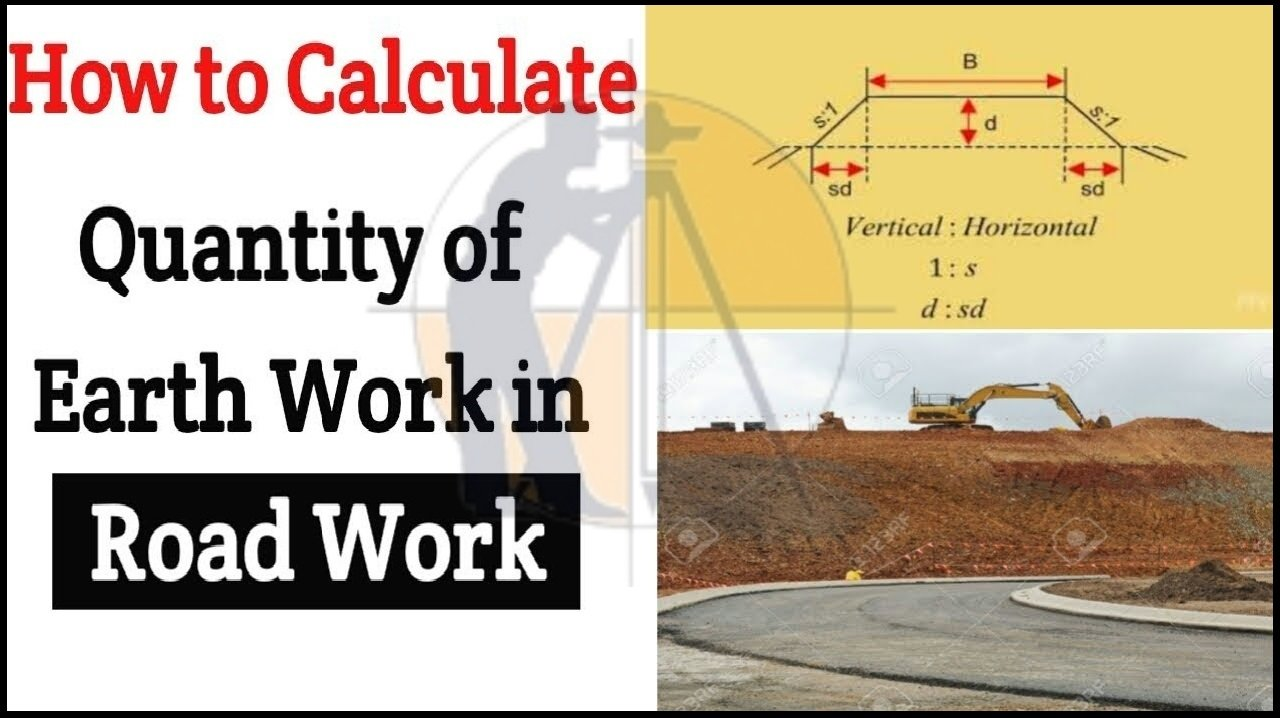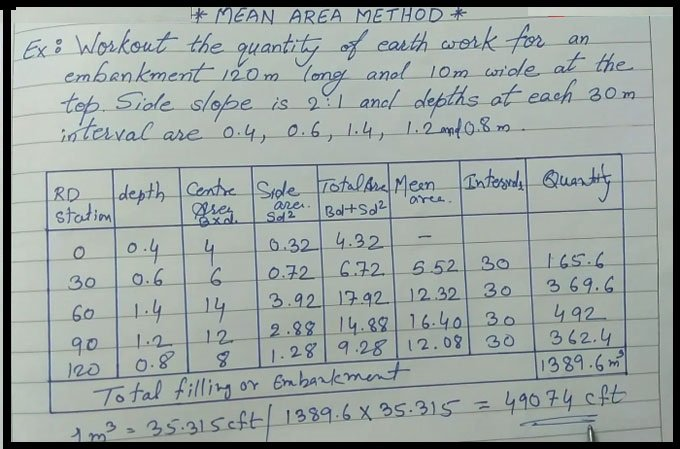Breaking News
Home / QA/QC Engineering / How to calculate the Quantity of Earthwork in the Road

# How to calculate the Quantity of Earthwork in the Road

### How to calculate the Quantity of Earthwork in Road.

In this Article u will learn step-by-step guidelines for measuring the QTY of earthwork (soil) in the Road, railway & the canal work with the help of mean area method.For explanation, the solution is given in the following example.

Example ? Calculate the QTY of the earthwork for an embankment with length 120 m and width ten m at the top. Side slope is given as 2:1 & depths at each Thirty m distance are 0.4, 0.6, 1.4, 1.2 and 0.8 m.

The calculation is the done through a table. The table contains various heads like Road station, depth, center area, side area, total area, mean area, intervals and Qty.

• ### Calculate the Volume And Weight of Asphalt For Road Construction

The work of the road will start from Zero point. So, in the road station column in the table, the values will be given as 0, 30, 60, 90, 120 (as distance is given as thirty m).In the depth column, provide the values as 0.4, 0.6, 1.4, 1.2 & 0.8 m.

To calculate the center area, the following formula will be used.

B x d = Breadth or width x Depth

After putting the values, we get the following result.

10 x 0.4 = fore meter
In this way the other values can be determined easily.

### To the determine side area, the following formula will be used.

Sd2, here S denotes slope & d denotes depth.

### After putting the values, we get the following output.

Two x 0.42 = 0.32 m2 (as sloe is given by 2:1)

In this way, the other values can be determined easily.

The Total area will be calculated with the following formula.

Bd + Sd2 = Center Area + Side area

To determine the mean area, 1st, sum up the first 2 rows of total area & then divide it with Two i.e. 4.32 + 6.72 /Two = 5.52 meter. In this way, other values can be calculated.

The value of intervals is given as Thirty meter.

### Finally, the total area will be calculated as follow.

Mean area x Intervals.

After putting the values, we get the following result.

5.52 x 30 = 165.6 m2

In this way, the other values can be calculated.

Total filling or embankment will be determined by summing up all the Qty & it will be 1389.6 m3

So, the total QTY of soils for filling or embankment of the road = 1389.6 m3.

### THANKS.